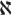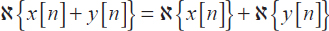## 6.2. Solved exercises

EXERCISE 6.1.

1. Write a MATLAB code to verify the linearity of system, represented by the transfer function:Consider for this two different signals x and y and demonstrate the following relationship:.

clf; n = 0:40; a = 2;b = -3;
x1 = cos(2*pi*0.1*n); x2 = cos(2*pi*0.4*n); x = a*x1 + b*x2;
num = [2.2403 2.4908 2.2403]; den = [1 -0.4 0.75];
ic = [0 0]; % set the initial conditions to zero
y1 = filter(num,den,x1,ic); %Calculation of the output signal y1[n]
y2 = filter(num,den,x2, ic); %Calculation of the output signal y2[n]
y = filter(num,den,x,ic); %Calculation of the output signal y[n]
yt = a*y1 + b*y2; d = y - yt; %Calculation of the error signal d[n]
% Plotting the error and the output signals
subplot(3,1,1); stem(n,y); ylabel('Amplitude');
title('Output corresponding to: a\cdot x_{1}[n] + b\cdot x_{2} [n]');
subplot (3,1,2); stem(n,yt) ; ylabel('Amplitude');
title('Output calculated as: a\cdot y_{1}[n] + b\cdot y_{2} [n]');
subplot(3,1,3); stem(n,d); xlabel('Time index n');
ylabel('Amplitude'); title('Error signal');Figure 6.1. Linearity of the convolution ...

Get Digital Signal Processing Using Matlab now with the O’Reilly learning platform.

O’Reilly members experience live online training, plus books, videos, and digital content from nearly 200 publishers.